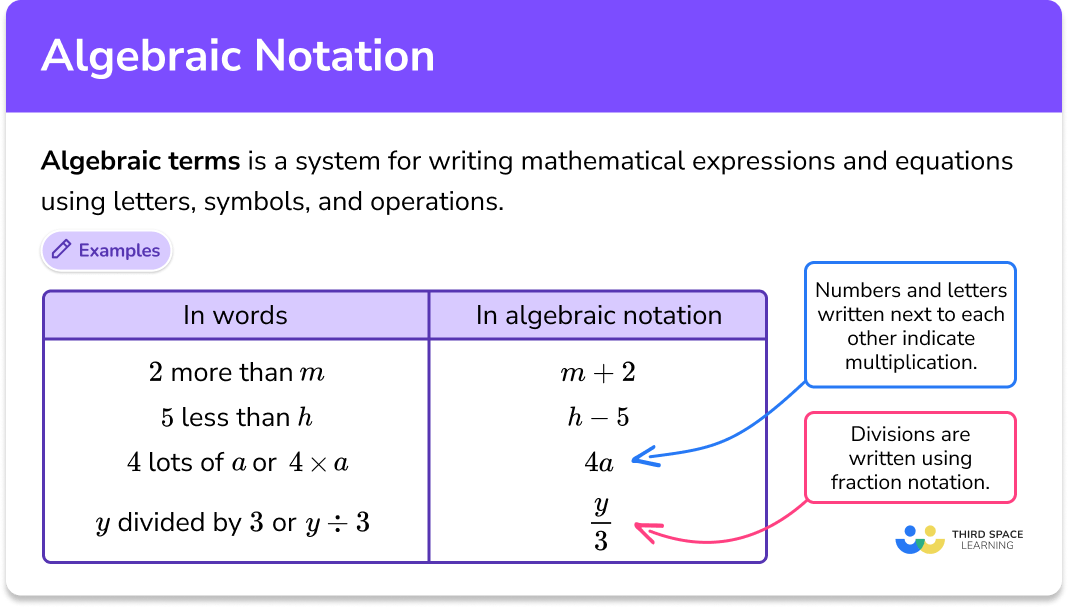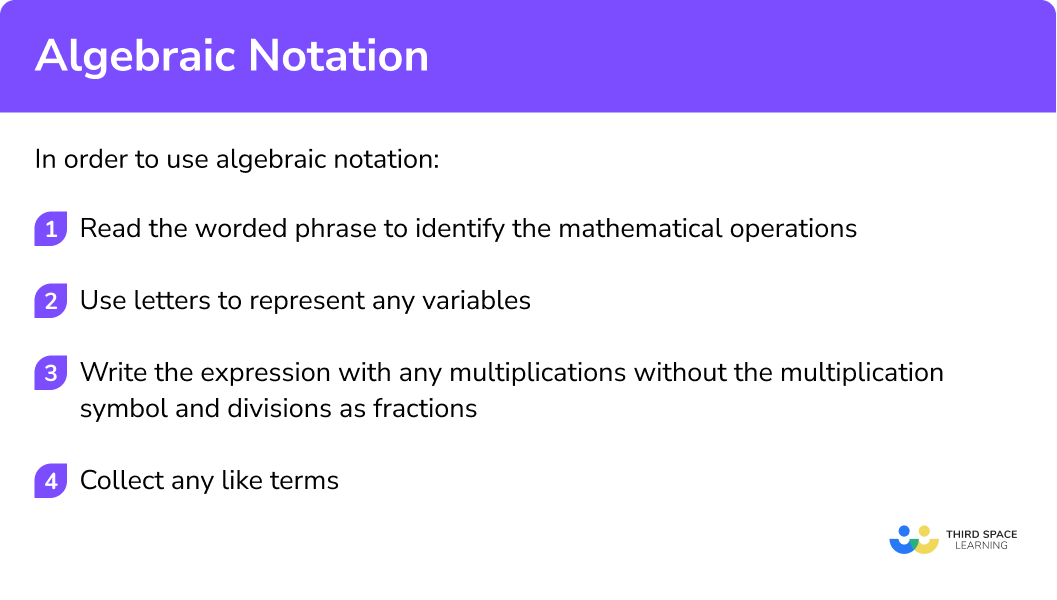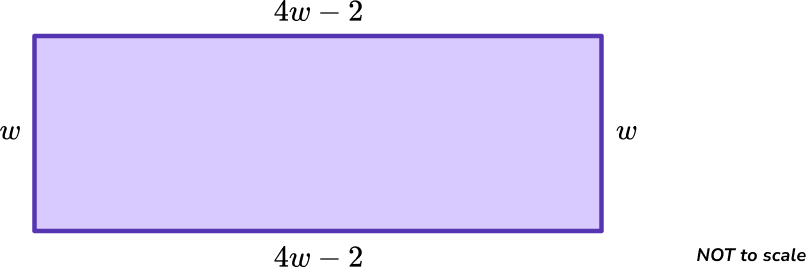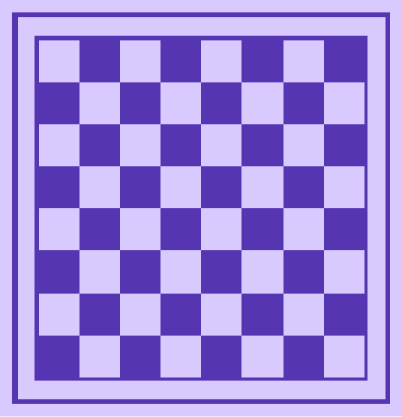# Algebraic Notation

Here we will learn about algebraic notation, including writing expressions and forming equations.

There are also worksheets based on Edexcel, AQA and OCR exam questions, along with further guidance on where to go next if you’re still stuck.

## What is algebraic notation?

Algebraic notation is a system for writing mathematical expressions and equations using letters, symbols, and operations.

It can be used to solve problems posed in worded form or added to the annotation of a diagram in geometrical problems to make a solution easier to find. Writing expressions using algebraic notation is a skill vital for GCSE Mathematics.

To do this we must first understand how to convert a mathematical expression in word form into algebraic notation. We need to know how to apply algebraic notation to the operations of addition, subtraction, multiplication and division.

The phrase “ 2 more than m ” can be written as m+2.

5 less than h ” can be written as h-5.

a less than 3 ” can be written as 3-a.

In algebra, when numbers and letters are written next to each other it indicates that they are multiplied together.

For example,

We write 4 \times a as 4a.

Multiplication is commutative so 4 \times a is the same as a \times 4, but that does not mean we write a4. When using algebraic notation for multiplication we always put the numerical coefficient before the letter.

When letters, or numbers and letters, are being divided, they are written in fraction form.

For example,

y \div 3 would be written in algebraic notation as \, \cfrac{y}{3}.

So if we had a problem that said the length of a rectangle is one more than 3 times the width. We could write l=3w+1, where l is the length and w is the width.

Algebraic notation is used across mathematics and science. In GCSE Mathematics and GCSE Science you will see many algebraic expressions and formulae.

Across both subjects you will use algebraic notation when plotting graphs, solving equations, inequalities, expanding brackets, factorising, simplifying expressions or algebraic fractions.

### What is algebraic notation?## How to use algebraic notation

In order to use algebraic notation:

1. Read the worded phrase to identify the mathematical operations.
2. Use letters to represent any variables.
3. Write the expression with any multiplications without the multiplication symbol and divisions as fractions.
4. Collect any like terms.

### Explain how to use algebraic notation## Algebraic notation examples

### Example 1: writing a sum using algebraic notation

A triangle has side lengths of 3 \ cm, 6 \ cm and x \ cm.

Write an expression for the perimeter of the triangle.

1. Read the worded phrase to identify the mathematical operations.

The perimeter of a shape is found by finding the sum of the sides. We need to add the side lengths together.

2Use letters to represent any variables.

We already have been told that the variable is called x.

3Write the expression with any multiplications without the multiplication symbol and divisions as fractions.

There are no multiplications or divisions, just additions.

3+6+x

4Collect any like terms.

We can collect the 3 and 6 to give the perimeter as

9+x.

### Example 2: writing a subtraction using algebraic notation

Sally is h \ cm tall. Her little brother Peter is shorter than Sally. Peter is 130 \ cm tall.

Write an expression for the difference in their heights.

Read the worded phrase to identify the mathematical operations.

Use letters to represent any variables.

Write the expression with any multiplications without the multiplication symbol and divisions as fractions.

Collect any like terms.

### Example 3: writing a product using algebraic notation

A rectangle has a length of 5 \ cm and a width of y \ cm.

Write an expression for the area of the rectangle.

Read the worded phrase to identify the mathematical operations.

Use letters to represent any variables.

Write the expression with any multiplications without the multiplication symbol and divisions as fractions.

Collect any like terms.

### Example 4: writing a division using algebraic notation

Amrit has a bag of counters. He knows one quarter of the counters are blue counters in the bag but does not know how many counters there are in total.

Write an expression for the number of blue counters.

Read the worded phrase to identify the mathematical operations.

Use letters to represent any variables.

Write the expression with any multiplications without the multiplication symbol and divisions as fractions.

Collect any like terms.

### Example 5: forming an equation using algebraic notation

A triangle has three angles. The middle sized angle is 10^{\circ} more than the smallest angle. The largest angle is 30^{\circ} less than double the smallest angle.

Form an equation that could be used to find the size of the smallest angle.

Read the worded phrase to identify the mathematical operations.

Use letters to represent any variables.

Write the expression with any multiplications without the multiplication symbol and divisions as fractions.

Collect any like terms.

### Example 6: writing a formula using algebraic notation

To find the original price of an item in a sale, we can divide the sale price by the percentage remaining if written as a decimal or fraction.

Write a formula to find the original price O of an item in a p\% sale with a sale price S.

Read the worded phrase to identify the mathematical operations.

Use letters to represent any variables.

Write the expression with any multiplications without the multiplication symbol and divisions as fractions.

Collect any like terms.

### Common misconceptions

• Not putting numerical coefficients in front of the letter

It is important to write h\times 8 as 8h and not h8.

• Not using powers correctly

A common error is to write a\times a\times a as 3a, when it should be a^3.

• Writing a subtraction in the wrong order

6 less than x is x-6 and not 6-x.

### Practice algebraic notation questions

1. Write “ 4 more than y ” using algebraic notation.

4-yy+44yy-44 more” involves adding 4, so we add 4 onto y to make the expression y+4.

2. Write “ 9 less than x ” using algebraic notation.

9-xx-99+x\cfrac{x}{9}9 less” involves subtracting 9, so we subtract 9 from x to make the expression x-9.

3. Write “ g less than 6 ” using algebraic notation.

6-g\cfrac{6}{g}g-66+gg less” involves subtracting g, so we subtract g from 6 to make the expression 6-g.

4. Write “ 1 less than 2 times x ” using algebraic notation.

\cfrac{2}{x}-11-2xx^2 -12x-12 times x ” is written as 2x. 1 less” means subtracting 1, so we subtract 1 from 2x to make the expression 2x-1.

5. A rectangle has a length which is 2 \ cm less than 4 times its width. Write an expression for the perimeter of the rectangle.

10w-45w-24w-410w+4Let w be the width of the rectangle.

The length of the rectangle will be 4w-2.The perimeter will be the sum of all 4 sides of the rectangle,

w+4w-2+w+4w-2.

Collecting the like terms will simplify this expression to

10w-4.

6. The price of 2 apples and 3 bananas is £1. The price of 4 apples and 7 bananas is £2.20. Write this information as a pair of simultaneous equations where a is the cost of one apple and b is the cost of one banana.

a^2+b^3=1, \; a^4+b^7=2.2a2+b3=1, \; a4+b7=2.22a+3b=1, \; 4a+7b=2.26ab=1, \; 28ab=2.2The first piece of information can be written as

2a+3b=1.

The second piece of information can be written as

4a+7b=2.2.

These can be solved as simultaneous equations to give a=0.2 and b=0.2.

This means that the cost of one apple is 20p and the cost of one banana is also 20p.

### Algebraic notation GCSE questions

1. Each small square on a chessboard used in a chess game has a side length of x \ cm.(a) Write an expression for the perimeter of the chessboard.

(b) Write an expression for the area of the chessboard.

(4 marks)

(a)

Side length = 8x

(1)

Perimeter = 32x

(1)

(b)

8x \times 8x

(1)

64x^2

(1)

2. The width of a rectangle is 1 \ cm less than half its length.

(a) Write an expression for the perimeter of the rectangle if its length is l \ cm.

(b) Given that the perimeter of the rectangle is 22 \ cm, find its area.

(7 marks)

(a)

\cfrac{l}{2}-1

(1)

\cfrac{l}{2}-1+\cfrac{l}{2}-1+l+l

(1)

3l-2

(1)

(b)

3l-2=22

(1)

l=8

(1)

8\times (\cfrac{8}{2}-1)

(1)

24 \ cm^2

(1)

3. To give the correct dose of a medicine (in mg ) to a child, the following steps must be taken.

Divide the child’s mass (in kg ) by 20, then add 15.

Write a formula for the dose, D, of a child of mass, m \ kg.

(3 marks)

D =

(1)

\cfrac{m}{20}

(1)

D=\cfrac{m}{20}+15

(1)

## Learning checklist

You have now learned how to:

• Form expressions and equations using algebraic notation
• Identify algebraic terms and state their variables and coefficients

## Still stuck?

Prepare your KS4 students for maths GCSEs success with Third Space Learning. Weekly online one to one GCSE maths revision lessons delivered by expert maths tutors.

Find out more about our GCSE maths tuition programme.